# Range

This is the current revision of Range as edited by at 06:32, 12 July 2016. This URL is a permanent link to this version of this page.

(diff) ← Older revision | Latest revision (diff) | Newer revision → (diff)
Jump to: navigation, search

In mathematics, the range (or image) of a function are the values it hits. It is not to be confused with the codomain of a function, which is a designated set to which all the values of the function belong.

A function is onto (or surjective) if every value in its codomain is hit by the function, or, equivalently, if its range is equal to its codomain. More formally, a functionis onto if for every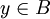there existssuch that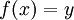.

## Examples

Letbe the function defined by the equation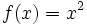. By definition, the codomain of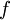is. However, the range ofconsists of all nonnegative real numbers. Indeed, letbe a nonnegative real number. Then, and sois one of the values hit by.

Letbe the function defined by the equation. Then, for every real number, we can see that, so every real number is hit by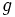. This means that the codomain and range ofare equal, namely. Therefore,is onto.